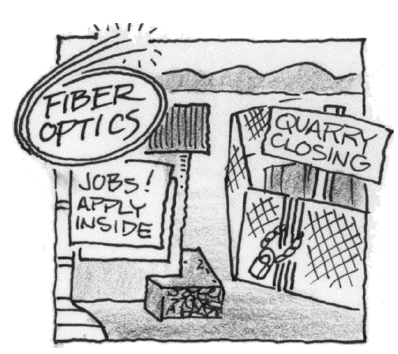### Home > CALC > Chapter 6 > Lesson 6.2.2 > Problem6-76

6-76.

The good news for the small prairie town of Woisme is that a new fiber optics plant is about to open. The bad news is that the old quarry is about to close, and many residents will lose their jobs as a result. The population is predicted to follow this model over the next few years:
$p(t) = 100(4 · 2^t − 3^t ) = 400 · 2^t − 100 · 3^t$ .

1. Using complete sentences, relate the equation for $p(t)$ to the changes of industry.

There are both positive and negative components to $p(t)$. What do you think these components correspond to?

2. Algebraically determine when Woisme will have the most people. Use your calculator only for numerical calculations.

Find $p^\prime(t)$ and determine when $p^\prime(t) = 0$.

Be sure to consider the endpoints. For example, Woisme's population might have been the greatest at the $t = 0$.

$≈ 2.283$ years